## Example Questions

### Example Question #1 : How To Use The Direct Variation Formula

The price of silver varies directly as the square of the mass. If 3.6 g of silver is worth $64.80, what is the value of 7.5 g of silver? Possible Answers:$135.00

$301.75$178.50

$215.25$281.25

$281.25 Explanation: This is a direct variation problem of the form y = kx2 The first set of data 3.6 g and$64.80 is used to calculate the proportionality constant, k.  So 64.80 = k(3.6)2 and solving the equation gives k = 5.

Now we move to the new data, 7.5 g and we get y = 5(7.5)2 to yield an answer of $218.25.$135.00 is the answer obtained if using proportions.  This is an error because it does not take into consideration the squared elements of the problem.

### Example Question #2 : How To Use The Direct Variation Formula

The diameter of a specific brand of candy wrapper is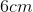longer than half the volume of the candy itself. Find the expression for the diameter,, in terms of the volume,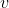.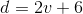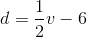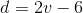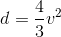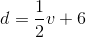Explanation:

The question asks for an equation that can relateandto each other, based on the information given. We are told that half the volume +determines the total diameter.

This gives us:### Example Question #3 : How To Use The Direct Variation Formula

An instrument reads two values,anddaily. The values directly vary with respect to each other. If on Monday the value ofwasandwas, which of the following could be the values forandon Wednesday?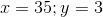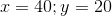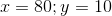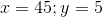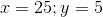Explanation:

An instrument reads two values,anddaily. The values directly vary with respect to each other. If on Monday the value ofwasandwas, which of the following could be the values forandon Wednesday?

Direct variation means that any pairing of the related values will always have the same ratio, thus we know that for any other valuesand, those values will be equal according to the following equation: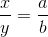Thus, for our information, we know: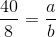This means that the new values ofand, when divided must be equal to. Therefore, the only possible answer is### Example Question #4 : How To Use The Direct Variation Formula

In an experimental setting, the pressure and the temperature of gas in a container are directly proportional. In the first experiment, the pressure of the gas was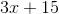and the temperature of the gas was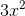, whereis a constant value not equal to. If a second experiment found a temperature of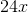, which of the following represents the pressure of the gas?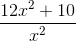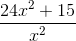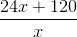It cannot be determined.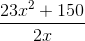Explanation:

For direct variation of related variables, we know that the following equation holds: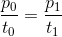For our data, this would be: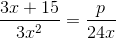To start simplifying and solving this, first factor the top of the left fraction: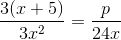Cancel thes: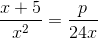Next, multiply by: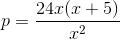Sincedoes not equal, cancel thes: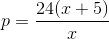Simplify: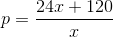### Example Question #5 : How To Use The Direct Variation Formula

In a given solution, the proportion of water to apple juice is directly proportional. If the first batch of the solution containedgallons of apple juice and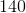gallons of water, how many gallons of apple juice will be needed for a solution containinggallons of apple juice?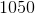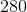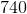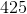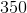Explanation:

This problem is easily solved by setting up a ratio. For directly proportional amounts, we know: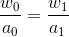For our data, this would be: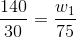This is first simplified as being: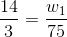Next, multiply byon both sides to solve for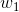: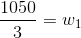Finally: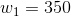### Example Question #6 : How To Use The Direct Variation Formula

The length, in inches, of a box is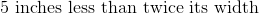. Which of the following gives the length,inches, in terms of the width,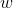inches, of the box?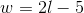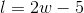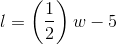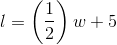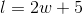Since the question asks for the length in terms of width, I like to start by setting the problem up as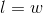, then add the conditions off the problem. I start at the first condition with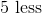than the width and go ahead and add a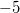after, then it sayless than TWICE the width, so I add myin front of the. This gives you,  essentially you're working the problem piece by piece.